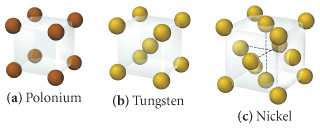# Problem: Determine the number of atoms per unit cell for each of the following metals .Polonium

###### FREE Expert Solution

We're asked to determine the number of atoms per unit cell for each of the following metals (a) polonium (b) tungsten (c) nickel

First, we need to determine the type of unit cell

•The Simple Cubic Unit Cell is composed of a cube with an atom at each corner

•The body-centered cubic unit cell is composed of a cube with one atom at each of its corners and one atom at the center of the cube

•The face-centered cubic unit cell is composed of a cube with one atom at each of its corners and one atom in the center of each cube face.

83% (304 ratings)###### Problem Details

Determine the number of atoms per unit cell for each of the following metals .Polonium